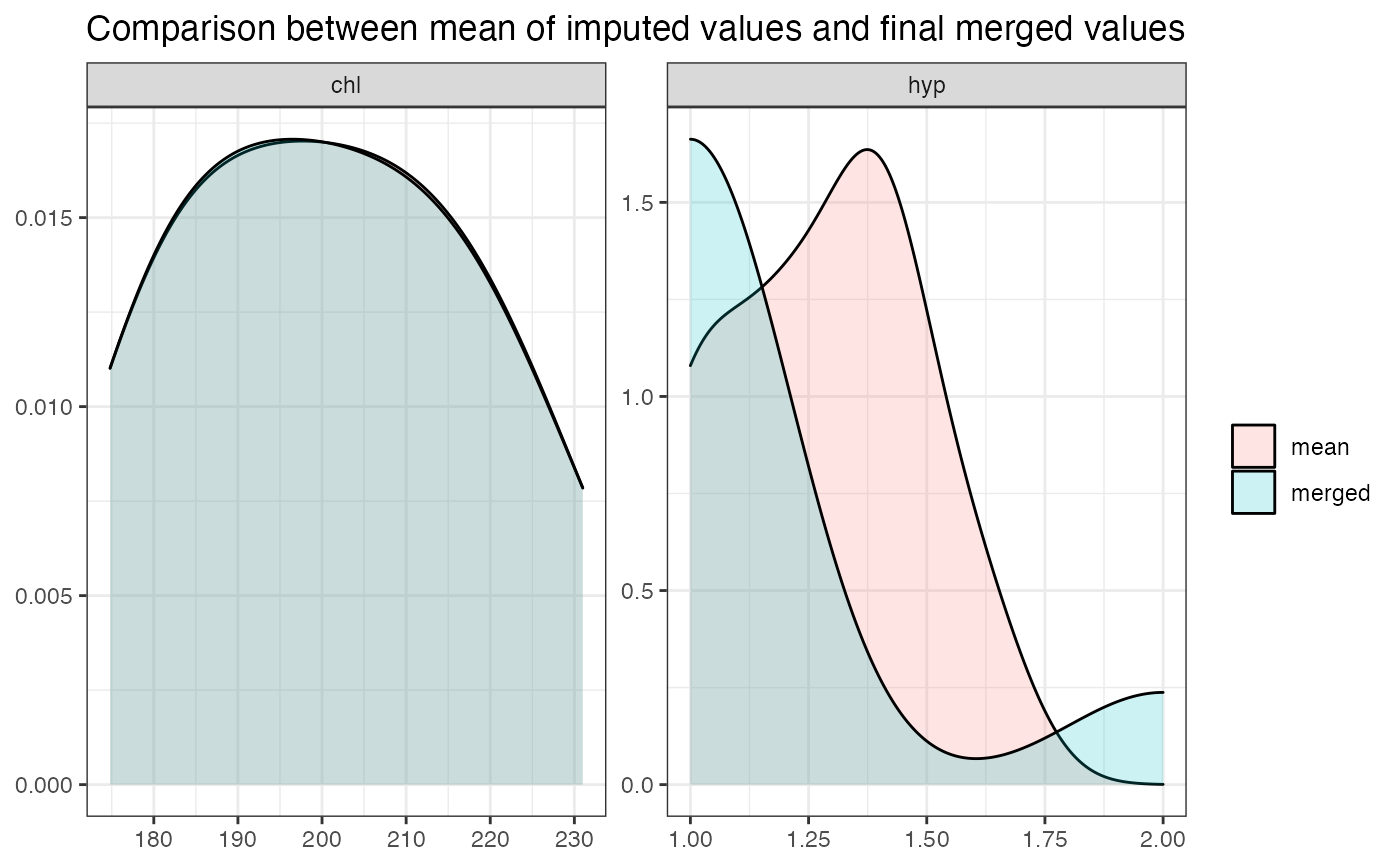This function merges multiple imputed data frames from mice::mids()-objects into a single data frame by computing the mean or selecting the most likely imputed value.

merge_imputations(
dat,
imp,
ori = NULL,
summary = c("none", "dens", "hist", "sd"),
filter = NULL
)

## Arguments

dat

The data frame that was imputed and used as argument in the mice-function call.

imp

The mice::mids()-object with the imputed data frames from dat.

ori

Optional, if ori is specified, the imputed variables are appended to this data frame; else, a new data frame with the imputed variables is returned.

summary

After merging multiple imputed data, summary displays a graphical summary of the "quality" of the merged values, compared to the original imputed values.

"dens"

Creates a density plot, which shows the distribution of the mean of the imputed values for each variable at each observation. The larger the areas overlap, the better is the fit of the merged value compared to the imputed value.

"hist"

Similar to summary = "dens", however, mean and merged values are shown as histogram. Bins should have almost equal height for both groups (mean and merged).

"sd"

Creates a dot plot, where data points indicate the standard deviation for all imputed values (y-axis) at each merged value (x-axis) for all imputed variables. The higher the standard deviation, the less precise is the imputation, and hence the merged value.

filter

A character vector with variable names that should be plotted. All non-defined variables will not be shown in the plot.

## Value

A data frame with (merged) imputed variables; or ori with appended imputed variables, if ori was specified. If summary is included, returns a list with the data frame data with (merged) imputed variables and some other summary information, including the plot as ggplot-object.

## Details

This method merges multiple imputations of variables into a single variable by computing the (rounded) mean of all imputed values of missing values. By this, each missing value is replaced by those values that have been imputed the most times.

imp must be a mids-object, which is returned by the mice()-function of the mice-package. merge_imputations() than creates a data frame for each imputed variable, by combining all imputations (as returned by the complete-function) of each variable, and computing the row means of this data frame. The mean value is then rounded for integer values (and not for numerical values with fractional part), which corresponds to the most frequent imputed value (mode) for a missing value. Missings in the original variable are replaced by the most frequent imputed value.

## Note

Typically, further analyses are conducted on pooled results of multiple imputed data sets (see pool), however, sometimes (in social sciences) it is also feasible to compute the mean or mode of multiple imputed variables (see Burns et al. 2011).

## Examples

if (require("mice")) {
imp <- mice(nhanes)

# return data frame with imputed variables
merge_imputations(nhanes, imp)

# append imputed variables to original data frame
merge_imputations(nhanes, imp, nhanes)

# show summary of quality of merging imputations
merge_imputations(nhanes, imp, summary = "dens", filter = c("chl", "hyp"))
}
#>
#> Attaching package: ‘mice’
#> The following object is masked from ‘package:stats’:
#>
#>     filter
#> The following objects are masked from ‘package:base’:
#>
#>     cbind, rbind
#>
#>  iter imp variable
#>   1   1  bmi  hyp  chl
#>   1   2  bmi  hyp  chl
#>   1   3  bmi  hyp  chl
#>   1   4  bmi  hyp  chl
#>   1   5  bmi  hyp  chl
#>   2   1  bmi  hyp  chl
#>   2   2  bmi  hyp  chl
#>   2   3  bmi  hyp  chl
#>   2   4  bmi  hyp  chl
#>   2   5  bmi  hyp  chl
#>   3   1  bmi  hyp  chl
#>   3   2  bmi  hyp  chl
#>   3   3  bmi  hyp  chl
#>   3   4  bmi  hyp  chl
#>   3   5  bmi  hyp  chl
#>   4   1  bmi  hyp  chl
#>   4   2  bmi  hyp  chl
#>   4   3  bmi  hyp  chl
#>   4   4  bmi  hyp  chl
#>   4   5  bmi  hyp  chl
#>   5   1  bmi  hyp  chl
#>   5   2  bmi  hyp  chl
#>   5   3  bmi  hyp  chl
#>   5   4  bmi  hyp  chl
#>   5   5  bmi  hyp  chl